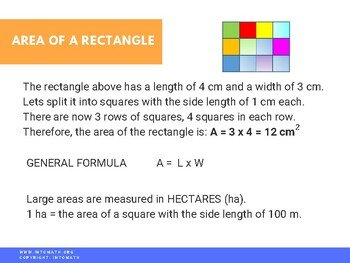CA$2.00 We are often required to calculate the area and perimeter of objects or spaces in 2 dimensions (flat surface like a piece of paper or a field). In real life, this skill is helpful when designing living spaces or calculating the amount of fencing required to enclose a certain piece of land, etc. Perimeter is the length and width around the object – the sum of all outer sides. Area – the 2 dimensional space surrounded by the sides of the shape.Grade 7 Workbook (22 pages with answers) CA$4.00

The two shapes we are looking at in this lesson are rectangle and square.

A square is also a rectangle, but a regular rectangle. “Regular” means all sides and angles are equal.

We develop formulas for both area and perimeter and analyze different situations where those formulas can be applied. It is important to not only memorize the formulas or substitute numbers into them. It is important to understand where the formulas are coming from, how they were derived. Making connections and understanding relationships is one of the key components of being successful in math.

### Perimeter and area short animation

#### Grade 7 Rectangle Area and Perimeter Quiz